# Chemical Reactions and Equations - NCERT Questions

Q 1.

Why should magnesium ribbon be cleaned before burning in air?

SOLUTION:

Ans : Magnesium ribbon is cleaned before burning so that coating of impurity (such as oxide) formed on its surface is removed and it becomes pure magnesium.

Q 2.

Write the balanced equations for the following chemical reactions.
(a) Hydrogen + chlorine → hydrogen chloride
(b) Barium chloride + aluminium sulphate → barium sulphate + aluminium chloride
(c) Sodium + water → sodium hydroxide + hydrogen

SOLUTION:

Ans :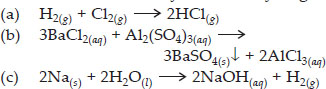Q 3.

Write a balanced chemical equation with state symbols for the following reactions :
(a) Solutions of barium chloride and sodium sulphate in water react to give insoluble barium sulphate and the solution of sodium chloride.
(b) Sodium hydroxide solution (in water) reacts with hydrochloric acid solution (in water) to produce sodium chloride solution and water

SOLUTION:

Ans :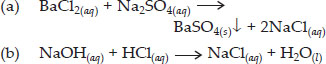Q 4.

A solution of the substance X is used for white washing.
(i) Name the substance X and write its formula.
(ii) Write the reaction of the substance X with water.

SOLUTION:

Ans : (i) The substance X is calcium oxide (also called quick lime). Its formula is CaO.
(ii)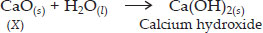Q 5.

Why is double the amount of gas collected in one of the test tubes in electrolysis of water than the amount collected in the other? Name the gas.

SOLUTION:

Ans : On electrolysis, water decomposes according to the reaction,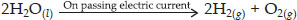Thus, water decomposes to give hydrogen and oxygen in the ratio 2 : 1 by volume. The double volume of the gas collected is hydrogen.

Q 6.

Why does the colour of copper sulphate solution change when an iron nail is dipped in it?

SOLUTION:

Ans : The reaction between copper(II) sulphate and iron is represented as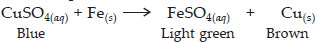In this displacement reaction, a more active element iron displaces another less reactive element from its compound copper(II) sulphate. The brown copper metal gets deposited on the iron nails. The colour of the solution changes from blue to light green due to the formation of Fe2+ ions.

Q 7.

Give an example of a double displacement reaction.

SOLUTION:

Ans : In the reaction of silver nitrate and sodium chloride, the silver exchanges its nitrate ions with chloride ions of sodium chloride and forms a precipitate of silver chloride. The chemical reaction is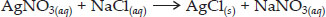Q 8.

Identify the substances that are oxidized and the substances that are reduced in the following reactions :
(i) 4Na(s) + O2(g) → 2Na2O(s)
(ii) CuO(s) + H2(g) → Cu(s) + H2O(l)

SOLUTION:

Ans :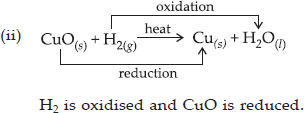Q 9.

Which of the statements about the reaction below are incorrect?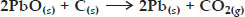(ii) Carbon dioxide is getting oxidised.
(iii) Carbon is getting oxidised.
(iv) Lead oxide is getting reduced.

 A. (i) and (ii) B. (i) and (iii) C. (i), (ii) and (iii) D. all of these

SOLUTION:

Ans : (A)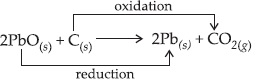Q 10.

Fe2 O 3 + 2Al → Al 2 O 3 + 2Fe The above reaction is an example of a

 A. combination reaction B. double displacement reaction C. decomposition reaction D. displacement reaction

SOLUTION:

Ans : (D) It is an example of displacement reaction.Fe of Fe2O3 has been displaced by Al.

Q 11.

What happens when dilute hydrochloric acid is added to iron filings? Tick the correct answer.

 A. Hydrogen gas and iron chloride are produced. B. Chlorine gas and iron hydroxide are produced. C. No reaction takes place. D. Iron salt and water are produced.

SOLUTION:

Ans : (A) Fe(s) + 2HCl(dil.) → FeCl2(aq) + H2(g)

Q 12.

What is a balanced chemical equation ? Why should chemical equations be balanced?

SOLUTION:

Ans : The chemical equation in which the number of atoms of each element on both the sides are equal is called the balanced equation.
A chemical equation should be balanced because there is no loss or gain of any matter during a chemical reaction, i.e., the law of conservation of matter must hold good for the reaction.

Q 13.

Translate the following statements into chemical equations and then balance them :

 A. Hydrogen gas combines with nitrogen to form ammonia. B. Hydrogen sulphide gas burns in air to give water and sulphur dioxide. C. Barium chloride reacts with aluminium sulphate to give aluminium chloride and a precipitate of barium sulphate. D. Potassium metal reacts with water to give potassium hydroxide and hydrogen gas.

SOLUTION: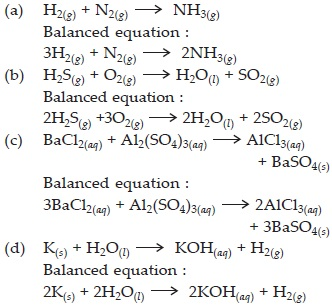Q 14.

Balance the following chemical equations :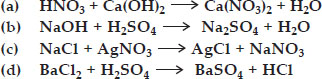SOLUTION: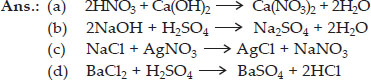Q 15.

Write the balanced chemical equations for the following reactions :

 A. Calcium hydroxide + Carbon dioxide → Calcium carbonate + Water B. Zinc + Silver nitrate → Zinc nitrate + Silver C. Aluminium + Copper chloride → Aluminium chloride + Copper D. Barium chloride + Potassium sulphate → Barium sulphate + Potassium chloride

SOLUTION: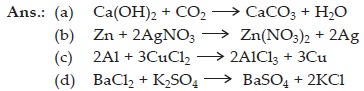Q 16.

Write the balanced chemical equations for the following and identify the type of reaction in each case.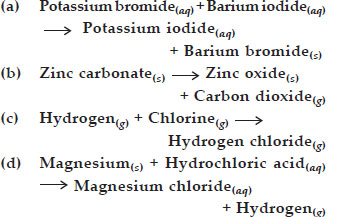SOLUTION: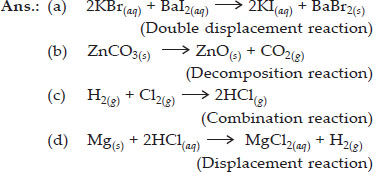Q 17.

What does one mean by exothermic and endothermic reactions? Give examples.

SOLUTION:

The chemical reactions which occur with the evolution of heat are called exothermic reactions.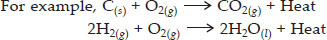The chemical reactions which occur with the absorption of heat are called endothermic reactions. For example,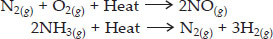Q 18.

Why is respiration considered as an exothermic reaction ? Explain.

SOLUTION:

Rice, potatoes and bread contain carbohydrates. During digestion, these carbohydrates are broken down into simpler substances called glucose. This glucose combines with oxygen in the cells of our body and provides energy. The special name of this reaction is respiration. Thus respiration is an exothermic process because energy is produced during this process.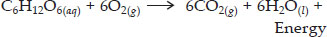Q 19.

Why are decomposition reactions called the opposite of combination reactions? Write equations for these reactions.

SOLUTION:

The decomposition reactions are those in which a compound breaks up into two or more simpler substances. On the other hand, combination reactions are those in which two or more substances combine to form single substance.
Thus, the decomposition reactions are opposite of the combination reactions.
For example, decomposition reactions are :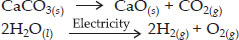For example, combination reactions are :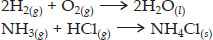Q 20.

Write one equation each for decomposition reactions where energy is supplied in the form of heat, light or electricity.

SOLUTION:

Decomposition reaction involving absorption of heat,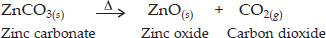Decomposition reaction involving absorption of light,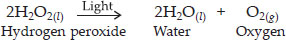Decomposition reaction involving absorption of electrical energy,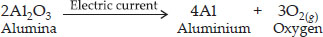Q 21.

What is the difference between displacement and double displacement reactions? Write equations for these reactions.

SOLUTION:

The chemical reactions in which one element takes the place of another element is called displacement reaction.
For example : Zinc displaces copper from copper sulphate solution :
Zn(s) + CuSO4(aq) → ZnSO4(aq) + Cu(s)
Double displacement reactions are those in which two compounds react to form two other compounds by mutual exchange of atoms or group of atoms. For example, in the reaction between barium chloride and sulphuric acid, barium chloride exchanges its Cl ions with SO42– ions of H2SO4.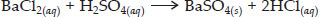Q 22.

In the refining of silver, the recovery of silver from silver nitrate solution involved displacement by copper metal. Write down the reaction involved.

SOLUTION: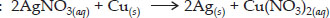Q 23.

What do you mean by a precipitation reaction? Explain by giving examples.

SOLUTION:

The reaction in which precipitates are produced are called precipitation reactions. For example,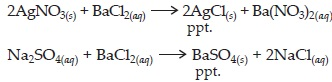Q 24.

Explain the following in terms of gain or loss of oxygen with two examples each.
(i) Oxidation (ii) Reduction

SOLUTION:

(i) Oxidation is a reaction which involves addition or gain of oxygen. For example,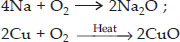(ii) Reduction is a reaction which involves loss or removal of oxygen. For example,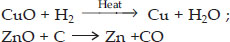Q 25.

A shiny brown coloured element 'X' on heating in air becomes black in colour. Name the element 'X' and the black coloured compound formed.

SOLUTION:

The element X is copper, Cu.
Black coloured coating is of copper oxide, CuO.

Q 26.

Why do we apply paint on iron articles?

SOLUTION:

We apply paint on iron articles so as to prevent it from rusting. When the surface of iron is coated with paint, its surface does not come in contact with oxygen and moisture, therefore, rusting does not take place.

Q 27.

Oil and fat containing food items are flushed with nitrogen.Why?

SOLUTION:

Oil and fats containing articles are flushed with nitrogen to prevent them from getting oxidised. This will protect them from becoming rancid.

Q 28.

Explain the following terms with an example each :
a. Corrosion
b. Rancidity

SOLUTION:

(A) Corrosion : It is a process of deterioration of metals as a result of its reaction with air or water present in environment. Therefore, when metals are exposed to atmosphere, they react with air or water present in the environment and form undesirable compounds on their surfaces. This process is called corrosion . Almost all metals except noble metals such as gold, platinum and palladium get corroded. For example, when iron is exposed to moisture for a long time, its surface acquires a brown flaky substance called rust. Similarly, surface of copper acquires a green coating of basic copper carbonate, etc.
(B) Rancidity : When fats and oils are exposed to air, they get oxidised and become rancid and their smell and colour change. This phenomenon is called rancidity. To prevent rancidity of food materials containing fats and oils, certain substances (called antioxidants) are added which prevent oxidation and hence rancidity. Some food materials are stored in air tight containers or the bags containing the food materials (such as chips) are flushed with inert gas such as nitrogen to prevent them from getting oxidised.